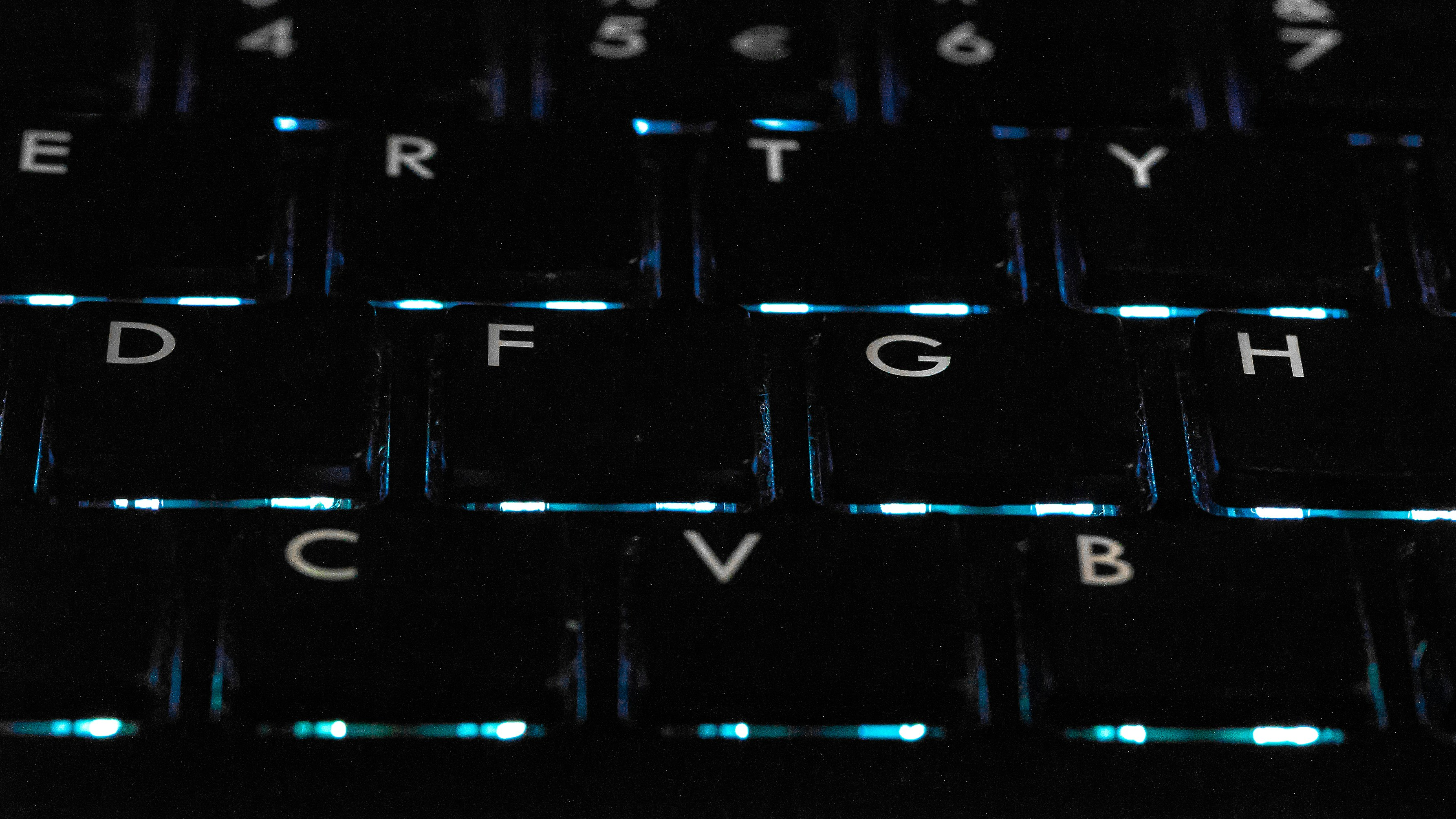# Assessing Grey Level of an Image with PHP``````<?php

/**
* Get gray level of some position of an image
*/
function get_gray_rgb(\$image, \$x, \$y) {
\$rgb  = imagecolorat(\$image, \$x, \$y);
//level of red, green and blue
\$red    = (\$rgb >> 16) & 0xFF;
\$green    = (\$rgb >> 8 ) & 0xFF;
\$blue    = \$rgb & 0xFF;

//formula to calculate gray level
\$gray = round(.299*\$red + .587*\$green + .114*\$blue);
return \$gray;
}

/**
* Calculate the average gray level of an area in an image
*/
function get_avg_gray(\$image, \$based_x, \$based_y, \$area_width, \$area_height, \$base_is_end=false) {
//	whether the based position is the beginning or the end
if (\$base_is_end) {
// when the based position is the end
\$ending_x    = \$based_x;
\$ending_y    = \$based_y;
// re-calculate the beginning point
\$based_x = \$based_x - \$area_width; \$based_y = \$based_y - \$area_height;
} else {
\$ending_x    = \$based_x+\$area_width;
\$ending_y    = \$based_y+\$area_height;
}
/*
* Sum up all gray levels/the number of pixels
* */
\$total_gray_level = 0;
for (\$i = \$based_x; \$i <= \$ending_x; \$i++) {
for (\$j = \$based_y; \$j <= \$ending_y; \$j++) {
\$total_gray_level += digital_crew_theme_get_gray_rgb(\$image, \$i, \$j);
}
}

return round(\$total_gray_level/((\$area_width+1)*(\$area_height+1)));
}

``````